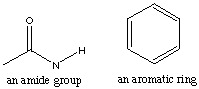1.* (1997 F 9) Identifying the useful spectroscopy. Hemocyanins, non-heme bimetallic copper proteins, are oxygen carriers in anthropods such as spiny lobster. Below the core structures of deoxy- and oxyhemocyanin are displayed. The protein which provides the histidine ligands is not shown. Deoxyhemocyanin has both copper ions in the +1 oxidation state. Oxy-hemocyanin is diamagnetic.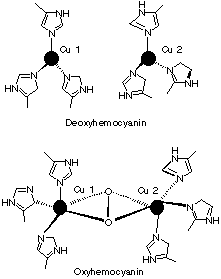A. Indicate the oxidation state of the copper ions and of the O2 ligand in oxyhemocyanin. B. What single method: mass spectrometry, UV-visible spectroscopy, NMR, microwave, or infrared/Raman spectroscopy, would be best suited to verify the oxidation state of the O2 ligand in oxyhemocyanin? Briefly explain your answer--for example, would oxygen isotopes such as 18O2 be useful? C. Indicate the number of electrons in the copper ions within both deoxy and oxy hemocyanin. D. Why do you think oxyhemocyanin is diamagnetic? Click here for solution to problem 1. 2. * (1997 F 16) Beer’s Law and kinetics. (NOTE: This problem contains both spectroscopy and kinetics. Before understanding kinetics, it’s not going to make much sense.) A cylindrical tube of length l and radius r is placed between a light source of wavelength l and a light detector.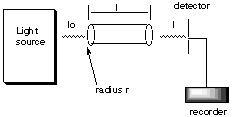The tube is filled with water and the transmitted intensity is found to be Io. Then x moles of A are added to the water in the tube. Compound A completely dissovles in water and undergoes a bimolecular reaction to form the dimer A2 which absorbs light at wavelength l with an extinction coefficient e. Compound A is transparent at wavelength l. The rate constant for the dimerization step is kd. Derive an expression for how the transmitted intensity at wavelength l changes with time, i.e. find an expression for I(t). Click here for solution to problem 2. 3.* (1997 2 5) Mass spectroscopy A. An unknown pure compound X has a mass spectrum in which the most prominent peak occurs at m/z = 86. Assume that X contains C and H but that O may or may not be present. Write down all possible molecular formulas for X that make chemical sense. B. Closer inspection shows that in addition to the ion at m/z = 86 there is a weaker mass peak at m/z = 87. The intensity ratio of the weaker to the stronger is 4.7% plus or minus 0.5 %, where the uncertainty represents a 99% confidence level. What can you conclude about the molecular formula for compound X? C. 1H NMR of compound X shows one peak. What are the chemically sensible structures for compound X? D. Further inspection of the mass spectrum for compound X shows a prominent peak at m/z = 71 and m/z = 43. Explain how these peaks come about. Click here for solution to problem 3. 4.* (1997 2 6) Infrared spectroscopy. What follows is the infrared spectrum of the compound C8H12. Assign those features you can in this spectrum and from these assignments suggest the structure of the C8H12 compound. Explain your reasoning.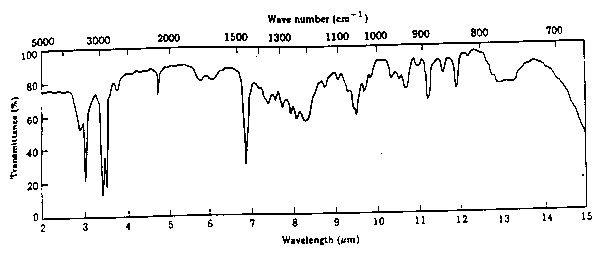Click here for solution to problem 4. 5. *(1996 2 7) Uv-vis spectroscopy. The following is a schematic diagram of an instrument designed to make absorption measurements: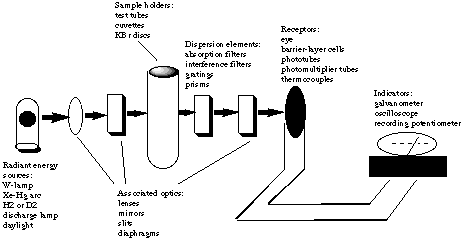A. The molar absorbance e of Br2 is 368.5 L mol-1 cm-1 at 400 nm. A cell 2 cm long is filled with solution of Br2 of unknown concentration. It is found that 60% of the 400 nm light incident upon it is absorbed. Calculate the concentration of Br2 in this solution. Be sure to state your answer with its units. B. A reagent is added to this solution of Br2 that reacts with it to form compounds that do not absorb at 400 nm. As the reaction proceeds, the Br2 is destroyed. Suppose that the rate of Br2 destruction is exponential in time, that is, the concentration c(t) at time t is related to the initial concentration c(0) at time t=0 by c(t) = c(0) exp (-kt) where k is the rate constant. Note that if the time is measured in seconds, then the units of k are inverse seconds. For our solution the absorption of the incident 400 nm light goes from 60% to 20% in 8 seconds after the time the reagent is added to the Br2 solution. Use this information to determine the value of k. C. Discuss whether it was necessary to know the values of the absorbance, the path length, or the initial concentration of the Br2 soluction to find the value of k. D. Suppose a chiral compound of limited amount has been synthesized. You are asked to determine if the synthesis was successful, that is, whether the compound has a large excess of one enantiomer over the other or is a racemic mixture. Explain how the following setup might be used to accomplish this task. Describe how this device works.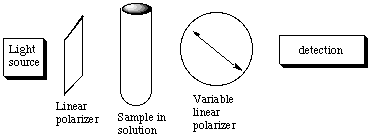E. For weakly absorbing samples, it may be advantageous to place the sample between two highly reflecting mirrors. Explain how this device increases the sensitivity of the absorption meausrement.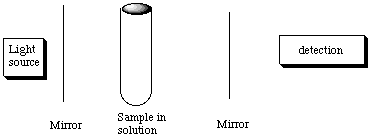F. It might seem natural to combine these two setups: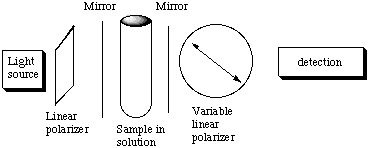Actually, this combination is a poor idea. Explain why the above setup is essentially without merit for increasing the sensitivity for measureing optical activity. Click here for solution to problem 5. 6.* (1995 2 6) Uv-vis spectroscopy. A radioactive compound A is dissolved in water to form a solution with initial concentreation c0 at time t = 0. Some of this solution is used to fill a cuvette that is placed inside a single-beam UV-vis spectrophotometer. The wavelength of the spectrophotometer is set at a wavelength at which the compound A absorbs. As time passes, the radioactive compound A decays according to the first-order rate expression c(t) = c0 e(-kt) where k is the rate constant for radioactive decay. Explain how to use Beer's law (I = Ioe(-ecl)) to find k. Discuss whether it is necessary to know the length l of the cuvette or the absorption coefficient e of compound A at the wavelength setting of the spectrophotometer. Click here for solution to problem 6. 7.* (1994 F 9) Visible "spectroscopy". An enzyme EH when placed in water undergoes the reaction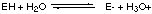in which equilibrium is rapidly attained and in which equilibrium lies far to the right, i.e. more [E-] than [EH]. A. Write down an expression for the equilibrium constant K for this reaction and show how the concentration ratio [E-] / [EH] depends on pH, i.e. on [H3O+]. For this particular enzyme the E- form is inactive whereas the form EH rapidly catalyzes the conversion of a substrate molecule S into the products P- and [H3O+], i.e.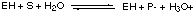Suppose at time t = 0 a neutral aqueous solution of this enzyme is placed inside a bag that is itself immersed in a neutral solution of the substrate S held in a container:The walls of the bag will not let EH or E- pass through them but are permeable to all other species. Initially, S starts to diffuse into the solution of EH and E- inside the bag. This diffusion process is slow. As S slowly diffuses into the bag, it reacts only with the EH form of the enzyme. At first the reaction rate is small because [EH] is small compared to [E-], but as products are formed (more rapidly than they can diffuse from the bag), [H3O+] increases inside the bag and shifts the rapidly attained enzyme equilibrium to make [EH] increase, which speeds up the reaction with S until a point is reached where S is consumed by reaction more rapidly than it can diffuse into the bag. Following this point P- and H3O+ must diffuse from higher concentration to lower concentration through the bag walls so that the enzyme concentration shifts to the right again lowering [EH] and returning to our starting point. B. Make a rough plot of [H3O+] versus time inside this diffusion-controlled bag (cell) reactor. C. Suggest how the [H3O+] concentration variation with time could be visually monitored inside the bag. D. Suppose the product P- has a characteristic UV absorption feature that is different from the UV absorption spectrum of S. Explain how the concentration of P- in the solution inside the container but outside the bag might be monitored in time. Click here for solution to problem 7. 8.* (1994 2 5) Shown in the figure below is a typical absorption spectrophotometer.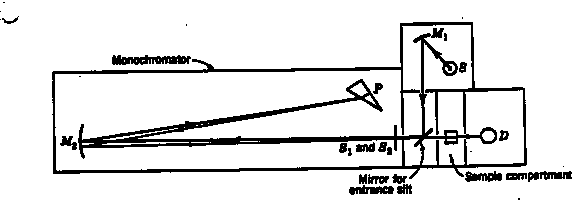The source is a battery-operated tungsten lamp that provides a "white" light for operation in the visible. A mirror M1 sends a beam of light from this source through the entrance slit S1 into the monochromator. This beam is reflected to a quartz prism by the collimating mirror M2. The prism P is of the so-called Littrow type so that the light undergoes refraction on entering the front surface, is relected from the solvered back surface, and undergoes a second refraction on leaving the prism. When the prism is rotated, a narrow band of light, which is nearly monochromatic at any desired wavelength in the visible, can be passed throught the exit slit S2. A sample cell, with plane parallel windows, is mounted in the sample compartment between the exit slit and the photodetector D. All light that is transmitted by the sample falls on the detector whose photocurrent is amplified and recorded. The magnitude of the photocurrent is proportional to the intensity of the transmitted light.

a. Suppose you were given an aqueous solution of Ru(bipy)32+ of unknown concentration [x] in units of moles/liter. Suppose also that you had available 10 g of pure Ru(bipy) 32+. Carefully explain what operations you would undertake to determine the value of [x]. Some possibly useful information follows: For Beer's law, I = Ioe(-ecl); Ru(bipy) 32+ has the chemical formula Ru(C10H8N2) 3, and the atomic weights are Ru = 101.07 g/mole, C = 12.011 g/mole, H = 1.008 g/mole, and N = 14.007 g/mole.

B. Prepare a set of instructions for a beginning student explaining how you would use the instrument shown in the figure to obtain an absorption spectrum of an aqueous solution of Ru(bipy)32+.

C. The ionic species tris (2,2’-bipyridine) ruthenium(II) Ru(bipy) 32+ has the structure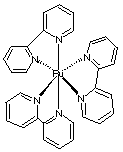and is chiral. Explain briefly how to resolve a racemic mixture of Ru(bipy) 32+ into its two enantiomers, and how to make sure that your separation procedure has worked.

The exact enantiomer recovered can be determined by verifying which direction the plane-polarized light is rotated (using a polarimeter) and comparing this to known experimental results.

9. * (1993 F B1E) IR absorption. The photochemical decomposition of HI in the gas phase at 266 nm results in the production of H2 and I2 in the gas phase. It is found experimentally that two molecules of HI are decomposed per photon absorbed. This information suggests two possible mechanisms in which the heat released is indicated for each step (recall that negative DH corresponds to positive heat release):

 Mechanism I DH (kcal/mol) hn + HI ----> H + I (fill in from part D) I + HI ----> H + I2 +35 H + H + M ----> H2 + M -104 Mechanism II DH (kcal/mol) hn + HI ----> H + I (fill in from part D) H + HI ----> H2 + I -33 I + I + M ----> I2 + M -36

In each mechanism M represents a third body that stabilizes the collision process.

Would it be possible to follow concentrations of reactants and products by infrared absorptions? Discuss.

10. * (1993 2 II2) Order the following types of radiation from longest wavelength on the top to shortest wavelength on the bottom: